# Title 40

## SECTION 53.35

### 53.35 Test procedure for Class II and Class III methods for PM 2.5 and PM −2.5.

§ 53.35 Test procedure for Class II and Class III methods for PM 2.5 and PM −2.5.

(a) Overview. Class II and Class III candidate equivalent methods shall be tested for comparability of PM 2.5 or PM 10-2.5 measurements to corresponding collocated PM 2.5 or PM 10-2.5 reference method measurements at each of multiple field sites, as required. Comparability is shown for the candidate method when simultaneous collocated measurements made by candidate and reference methods meet the comparability requirements specified in this section § 53.35 and in table C-4 of this subpart at each of the required test sites.

(b) Test sites and seasons. A summary of the test site and seasonal testing requirements is presented in table C-5 of this subpart.

(1) Test sites. Comparability testing is required at each of the applicable U.S. test sites required by this paragraph (b). Each test site must also meet the general test site requirements specified in § 53.30(b).

(i) PM2.5 Class II and Class III candidate methods. Test sites should be chosen to provide representative chemical and meteorological characteristics with respect to nitrates, sulfates, organic compounds, and various levels of temperature, humidity, wind, and elevation. For Class III methods, one test site shall be selected in each of the following four general locations (A, B, C, and D). For Class II methods, two test sites, one western site (A or B) and one midwestern or eastern site (C or D), shall be selected from these locations.

(A) Test site A shall be in the Los Angeles basin or California Central Valley area in a location that is characterized by relatively high PM 2.5, nitrates, and semi-volatile organic pollutants.

(B) Test site B shall be in a western city such as Denver, Salt Lake City, or Albuquerque in an area characterized by cold weather, higher elevation, winds, and dust.

(C) Test site C shall be in a midwestern city characterized by substantial temperature variation, high nitrates, and wintertime conditions.

(D) Test site D shall be in a northeastern or mid-Atlantic city that is seasonally characterized by high sulfate concentrations and high relative humidity.

(ii) PM10-2.5 Class II and Class III candidate methods. Test sites shall be chosen to provide modest to high levels of PM 10-2.5 representative of locations in proximity to urban sources of PM 10-2.5 such as high-density traffic on paved roads, industrial sources, and construction activities. For Class III methods, one test site shall be selected in each of the four following general locations (A, B, C, and D), and at least one of the test sites shall have characteristic wintertime temperatures of 0 °C or lower. For Class II methods, two test sites, one western site (A or B) and one midwestern or eastern site (C or D), shall be selected from these locations.

(A) Test site A shall be in the Los Angeles basin or the California Central Valley area in a location that is characterized by relatively high PM 2.5, nitrates, and semi-volatile organic pollutants.

(B) Test site B shall be in a western city characterized by a high ratio of PM 10-2.5 to PM 2.5, with exposure to windblown dust, such as Las Vegas or Phoenix.

(C) Test site C shall be in a midwestern city characterized by substantial temperature variation, high nitrates, and wintertime conditions.

(D) Test site D shall be in a large city east of the Mississippi River, having characteristically high sulfate concentrations and high humidity levels.

(2) Test seasons. (i) For PM 2.5 and PM 10-2.5 Class III candidate methods, test campaigns are required in both summer and winter seasons at test site A, in the winter season only at test sites B and C, and in the summer season only at test site D. (A total of five test campaigns is required.) The summer season shall be defined as the typically warmest three or four months of the year at the site; the winter season shall be defined as the typically coolest three or four months of the year at the site.

(ii) For Class II PM 2.5 and PM 10-2.5 candidate methods, one test campaign is required at test site A or B and a second test campaign at test site C or D (total of two test campaigns).

(3) Test concentrations. The test sites should be selected to provide ambient concentrations within the concentration limits specified in table C-4 of this subpart, and also to provide a wide range of test concentrations. A narrow range of test concentrations may result in a low concentration coefficient of variation statistic for the test measurements, making the test for correlation coefficient more difficult to pass (see paragraph (h) of this section, test for comparison correlation).

(4) Pre-approval of test sites. The EPA recommends that the applicant seek EPA approval of each proposed test site prior to conducting test measurements at the site. To do so, the applicant should submit a request for approval as described in § 53.30(b)(2).

(c) Collocated measurements. (1) For each test campaign, three reference method samplers and three candidate method samplers or analyzers shall be installed and operated concurrently at each test site within each required season (if applicable), as specified in paragraph (b) of this section. All reference method samplers shall be of single-filter design (not multi-filter, sequential sample design). Each candidate method shall be setup and operated in accordance with its associated manual referred to in § 53.4(b)(3) and in accordance with applicable guidance in “Quality Assurance Document 2.12” (reference (2) in appendix A to this subpart). All samplers or analyzers shall be placed so that they sample or measure air representative of the surrounding area (within one kilometer) and are not unduly affected by adjacent buildings, air handling equipment, industrial operations, traffic, or other local influences. The ambient air inlet points of all samplers and analyzers shall be positioned at the same height above the ground level and between 2 meters (1 meter for instruments having sample inlet flow rates less than 200 L/min) and 4 meters apart.

(2) A minimum of 23 valid and acceptable measurement sets of PM 2.5 or PM 10-2.5 24-hour (nominal) concurrent concentration measurements shall be obtained during each test campaign at each test site. To be considered acceptable for the test, each measurement set shall consist of at least two valid reference method measurements and at least two valid candidate method measurements, and the PM 2.5 or PM 10-2.5 measured concentration, as determined by the average of the reference method measurements, must fall within the acceptable concentration range specified in table C-4 of this subpart. Each measurement set shall include all valid measurements obtained. For each measurement set containing fewer than three reference method measurements or fewer than three candidate method measurements, an explanation and appropriate justification shall be provided to account for the missing measurement or measurements.

(3) More than 23 valid measurement sets may be obtained during a particular test campaign to provide a more advantageous range of concentrations, more representative conditions, additional higher or lower measurements, or to otherwise improve the comparison of the methods. All valid data sets obtained during each test campaign shall be submitted and shall be included in the analysis of the data.

(4) The integrated-sample reference method measurements shall be of at least 22 hours and not more than 25 hours duration. Each reference method sample shall be retrieved promptly after sample collection and analyzed according to the reference method to determine the PM 2.5 or PM 10-2.5 measured concentration in µg/m 3. Guidance and quality assurance procedures applicable to PM 2.5 or PM 10-2.5 reference methods are found in “Quality Assurance Document 2.12” (reference (2) in appendix A to this subpart).

(5) Candidate method measurements shall be timed or processed and averaged as appropriate to determine an equivalent mean concentration representative of the same time period as that of the concurrent integrated-sample reference method measurements, such that all measurements in a measurement set shall be representative of the same time period. In addition, hourly average concentration measurements shall be obtained from each of the Class III candidate method analyzers for each valid measurement set and submitted as part of the application records.

(6) In the following tests, all measurement sets obtained at a particular test site, from both seasonal campaigns if applicable, shall be combined and included in the test data analysis for the site. Data obtained at different test sites shall be analyzed separately. All measurements should be reported as normally obtained, and no measurement values should be rounded or truncated prior to data analysis. In particular, no negative measurement value, if otherwise apparently valid, should be modified, adjusted, replaced, or eliminated merely because its value is negative. Calculated mean concentrations or calculated intermediate quantities should retain at least one order-of-magnitude greater resolution than the input values. All measurement data and calculations shall be recorded and submitted in accordance with § 53.30(g), including hourly test measurements obtained from Class III candidate methods.

(d) Calculation of mean concentrations - (1) Reference method outlier test. For each of the measurement sets for each test site, check each reference method measurement to see if it might be an anomalous value (outlier) as follows, where Ri,j is the measurement of reference method sampler i on test day j. In the event that one of the reference method measurements is missing or invalid due to a specific, positively-identified physical cause (e.g., sampler malfunction, operator error, accidental damage to the filter, etc.; see paragraph (c)(2) of this section), then substitute zero for the missing measurement, for the purposes of this outlier test only.

(i) Calculate the quantities 2 × R1,j/(R1,j + R2,j) and 2 × R1,j/(R1,j + R3,j). If both quantities fall outside of the interval, (0.93, 1.07), then R1,j is an outlier.

(ii) Calculate the quantities 2 × R2,j/(R2,j + R1,j) and 2 × R2,j/(R2,j + R3,j). If both quantities fall outside of the interval, (0.93, 1.07), then R2,j is an outlier.

(iii) Calculate the quantities 2 × R3,j/(R3,j + R1,j) and 2 × R3,j/(R3,j + R2,j). If both quantities fall outside of the interval, (0.93, 1.07), then R3,j is an outlier.

(iv) If this test indicates that one of the reference method measurements in the measurement set is an outlier, the outlier measurement shall be eliminated from the measurement set, and the other two measurements considered valid. If the test indicates that more than one reference method measurement in the measurement set is an outlier, the entire measurement set (both reference and candidate method measurements) shall be excluded from further data analysis for the tests of this section.

(2) For each of the measurement sets for each test site, calculate the mean concentration for the reference method measurements, using equation 11 of this section: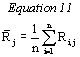Where: R j = The mean concentration measured by the reference method for the measurement set; Ri,j = The measurement of reference method sampler i on test day j; and n = The number of valid reference method measurements in the measurement set (normally 3).

(3) Any measurement set for which R j does not fall in the acceptable concentration range specified in table C-4 of this subpart is not valid, and the entire measurement set (both reference and candidate method measurements) must be eliminated from further data analysis.

(4) For each of the valid measurement sets at each test site, calculate the mean concentration for the candidate method measurements, using equation 12 of this section. (The outlier test in paragraph (d)(1) of this section shall not be applied to the candidate method measurements.)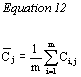Where: Cj = The mean concentration measured by the candidate method for the measurement set; Ci,j = The measurement of the candidate method sampler or analyzer i on test day j; and m = The number of valid candidate method measurements in the measurement set (normally 3).

(e) Test for reference method precision. (1) For each of the measurement sets for each site, calculate an estimate for the relative precision of the reference method measurements, RPj, using equation 13 of this section: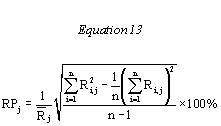(2) For each site, calculate an estimate of reference method relative precision for the site, RP, using the root mean square calculation of equation 14 of this section: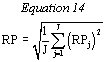Where, J is the total number of valid measurement sets for the site.

(3) Verify that the estimate for reference method relative precision for the site, RP, is not greater than the value specified for reference method precision in table C-4 of this subpart. A reference method relative precision greater than the value specified in table C-4 of this subpart indicates that quality control for the reference method is inadequate, and corrective measures must be implemented before proceeding with the test.

(f) Test for candidate method precision. (1) For each of the measurement sets, for each site, calculate an estimate for the relative precision of the candidate method measurements, CPj, using equation 15 of this section: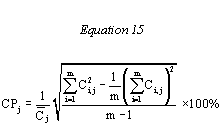(2) For each site, calculate an estimate of candidate method relative precision for the site, CP, using the root mean square calculation of equation 16 of this section: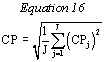Where, J is the total number of valid measurement sets for the site.

(3) To pass the test for precision, the mean candidate method relative precision at each site must not be greater than the value for candidate method precision specified in table C-4 of this subpart.

(g) Test for additive and multiplicative bias (comparative slope and intercept). (1) For each test site, calculate the mean concentration measured by the reference method, R , using equation 17 of this section: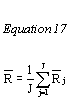(2) For each test site, calculate the mean concentration measured by the candidate method, C , using equation 18 of this section: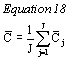(3) For each test site, calculate the linear regression slope and intercept of the mean candidate method measurements (C j) against the mean reference method measurements (R j), using equations 19 and 20 of this section, respectively: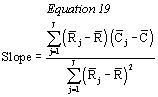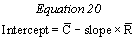(4) To pass this test, at each test site:

(i) The slope (calculated to at least 2 decimal places) must be in the interval specified for regression slope in table C-4 of this subpart; and

(ii) The intercept (calculated to at least 2 decimal places) must be in the interval specified for regression intercept in table C-4 of this subpart.

(iii) The slope and intercept limits are illustrated in figures C-2 and C-3 of this subpart.

(h) Tests for comparison correlation. (1) For each test site, calculate the (Pearson) correlation coefficient, r (not the coefficient of determination, r 2), using equation 21 of this section: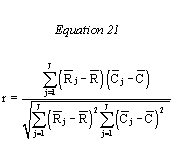(2) For each test site, calculate the concentration coefficient of variation, CCV, using equation 22 of this section: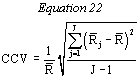(3) To pass the test, the correlation coefficient, r, for each test site must not be less than the values, for various values of CCV, specified for correlation in table C-4 of this subpart. These limits are illustrated in figure C-4 of this subpart.

[71 FR 61278, Oct. 17, 2006, as amended at 72 FR 32202, June 12, 2007]